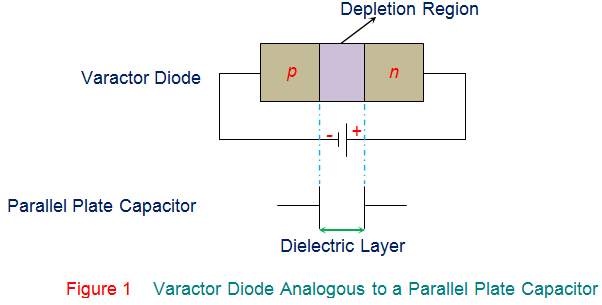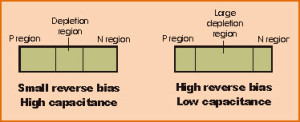# Theory of Varactor Diode Notes for Electronics Engineering 1st Year

## What is a Varactor Diode?

Varactor Diode is a reverse biased p-n junction diode, whose capacitance can be varied electrically. As a result these diodes are also referred to as varicaps, tuning diodes, voltage variable capacitor diodes, parametric diodes and variable capacitor diodes.

The varactor diode was named because of the variable reactor or variable reactance or variable capacitor or variable capacitance property of these diodes.## Varactor Diode Basics:

Even though the variable capacitance effect can be exhibited by the normal diodes (P-N junction diodes), but, varactor diodes are preferred for giving the desired capacitance changes as they are special types of diodes.

These diodes are specially manufactured and optimized such that they enables a very high range of changes in capacitance. Varactor diodes are again classified into various types based on the varactor diode junction properties. And, these are termed as abrupt varactor diodes, gallium arsenide varactor diodes, and hyper abrupt varactor diodes.

## Varactor Diode Symbol:

The symbol of varactor diode is shown in the figure. The varactor diode symbol consists of the capacitor symbol at one end of the diode that represents the variable capacitor characteristics of the varactor diodes.

in general, it looks like a normal PN- junction diode in which one terminal is termed as the cathode and the other terminal is termed as anode. Here, varactor diode consists of two lines at one end (cathode end of normal diode) that indicates the capacitor symbol.

## Varactor Diode Working:

To understand the working principle of the varactor diode, we must know what is a capacitor and how can we change the capacitance. Let us consider the capacitor that consists of two plates separated by an insulating dielectric as shown in the figure.

Consider the reverse biased mode of the diode, in which P-type region and N-type region are able to conduct and thus can be treated as two plates. The depletion region between the P-type and N-type regions can be considered as insulating dielectric. Thus, it is exactly similar to the capacitor shown above.

The size of the depletion region of diode changes with change in reverse bias. If the varactor diode reverse voltage is increased, then the depletion region size increases. Similarly, if the varactor diode reverse voltage is decreased, then the depletion region size decreases or narrows.Variation of Capacitance with Variation in Depletion Region of Varactor Diode

## Varactor Diode Characteristics:

The varactor diodes have the following significant characteristics:

• Varactor diodes produces considerably less noise compared to other conventional diodes.
• These diodes are available at low costs.
• Varactor diodes are more reliable.
• The varactor diodes are small in size and hence, they are very light weight.
• There is no useful purpose of varactor diode operated when it is operated in forward bias.
• Increase in reverse bias of varactor diode increases the capacitance as shown in the figure below.

## Varactor Diode Applications:

Varactor diodes finds large number of applications within the RF design arena and it is difficult to discuss about all the applications of varactor diodes or varicap diodes.

The capacitor in a circuit can be replaced with the varactor diode, but it is essential to ensure the tune voltage, i.e. the voltage required to set the varactor diode capacitance. And ensure that varactor diode is not affected by the bias voltage or any other voltages in the circuit. By using voltage control technique in the varactor diode circuit, varying capacitance can be provided.

## Voltage Controlled Oscillators (VCOs):

The  oscillator can be enabled by turning the diode D1. The capacitor C1 is used to prevent the reverse bias for the varactor diode, also avoids the diode getting short-circuited through the inductor. The varactor diode can be tuned by applying tuning voltage or bias through an isolating series resistor R1.

## RF Filters:

The varactor diodes can be used to tune the filters. In the receive front end circuits, tracking filters may be essential. The varactor diodes enable the filters to track the incoming received signal frequency which can be controlled using a control voltage. Usually, this is provided by microprocessor control through the digital to analog converter.

A few other important applications of varactor diodes can be listed as follows:

• Varactor diodes can be used as frequency modulators.
• In microwave receiver LO, varactor diodes can be used as frequency multipliers.
• Varactor diodes can be used as RF phase shifters.
• Varactor diodes are used to vary the capacitance in variable resonant tank LC circuits

### Feedback is important to us.

error: Content is protected !!